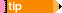Basic Structure of a Unit Test Basic Structure of a Unit Test Basic Structure of a Unit Test Basic Structure of a Unit Test Basic Structure of a Unit Test Basic Structure of a Unit Test Basic Structure of a Unit Test Basic Structure of a Unit Test Basic Structure of a Unit Test Basic Structure of a Unit Test Basic Structure of a Unit Test Basic Structure of a Unit Test Basic Structure of a Unit Test Basic Structure of a Unit Test Basic Structure of a Unit Test Basic Structure of a Unit Test Basic Structure of a Unit Test Basic Structure of a Unit Test Basic Structure of a Unit Test Basic Structure of a Unit Test Basic Structure of a Unit Test

### Basic Structure of a Unit Test

The following example shows the basic structure of a unit test:

The test starts with the [Isolated] attribute. This attribute ensures that the fakes are cleaned up after the test.

The test consists of the following stages:

Arrange, in which test preconditions are defined and test objects are set up

Act, in which the code under test is called

Assert, in which test results are inspected and failures are reported

A single point of entry is the Isolate statement. All mocking capabilities are available through this statement.

DateTime.Now() is faked and returns the date every time this method is called.You can find all code examples in the Examples folder installed with Typemock Isolator.

```C#

public class MyCode
{
public static int DoSomethingSpecialOnALeapYear()
{
if ((DateTime.Now.Month == 2) && (DateTime.Now.Day == 29))
return 100;
else
return 0;
}
}

[TestMethod, Isolated]
public void BasicUnitTestStructure()
{
// Arrange
Isolate.WhenCalled(() => DateTime.Now).WillReturn(new DateTime(2016, 2, 29));

// Act
int result = MyCode.DoSomethingSpecialOnALeapYear();

// Assert
Assert.AreEqual(100, result);
}
```

```VB

Public Class MyCode
Public Shared Function DoSomethingSpecialOnALeapYear() As Integer
If (DateTime.Now.Month = 2) AndAlso (DateTime.Now.Day = 29) Then
Return 100
Else
Return 0
End If
End Function
End Class

<TestMethod(), Isolated()> _
Public Sub BasicTestStructure()
' Arrange
Isolate.WhenCalled(Function() DateTime.Now).WillReturn(New DateTime(2016, 2, 29))

' Act
Dim result As Integer = My_Class.DoSomethingSpecialOnALeapYear()

' Assert
Assert.AreEqual(100, result)
End Sub
```